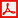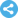American Journal of Mathematical and Computational Sciences
Manuscript Information

The Comparison of Entropy and Parameter Estimation in Complex Networks
American Journal of Mathematical and Computational Sciences
Vol.6 , No. 1, Publication Date: Jan. 22, 2021, Page: 1-5
144 Views Since January 22, 2021, 52 Downloads Since Jan. 22, 2021Paper in PDF (194K)Follow on

Authors

  Zhao Chunxue, School of Mathematics and Statistics, Anyang Normal University, Anyang, China.

Abstract

Entropy is used to describe the uniform distribution of any kind of energy in space. The more uniform the energy distribution, the higher the entropy. When the energy of a system is uniformly distributed, the entropy of the system reaches its maximum. Most complex systems can be described to network model, which includes a large number of nodes and complex connection relationships, Large number of networks show seemingly unrelated, but exist many striking similarities. According to different degree distribution, the network is divided into four kinds: regular network, random network, small-world network, scale-free network. Entropy is also a very important indicator which describes the heterogeneity of the networks. The scale-free network shows a non-homogeneous nature and a kind of sequence the complex network emerges. There have been some researches on using entropy to study complex networks. In the paper, we quantify the scale-free properties of the complex network by using the entropy theory and maximum likelihood estimation (MLE). We first review two kinds of entropy and prove their consistency; then we investigate the relationship of the parameter estimation among MLE, the moment estimator and the entropy of fitness scale-free network; finally, we gain the entropy of random network, which provides theoretical support for the practical application of entropy.

Keywords

Complex Network, Entropy, Maximum Likelihood Estimation, Moment Estimator

Reference

  S. Wasserman and K. Faust, Social network analysis: methods and applications. Cambridge, England: Canbridge University Press, 1994.  M. Faloutsos, P. Faloutsos and C. Faloutsos, “On power-law relationships of the internet topology,” Comput Commun Rev., 1999, 29, 251-262.  S. Lawrence and C. L. Giles, “Searching the world wide web,” Science, 1998, 280, 98-100.  P. Erdos and A. Renyi, “On random graphs,” Publicationes Mathematicae, 1959, 6, 290-297.  A. L. Barabasi, R. Albert and H. Jeong, “Mean-field theory for scale-free random networks,” Physica A, 1999, 272, 173-187.  R. Albert, H. Jeong and A. L. Barabasi, “Diameter of the world-wide web,” Nature, vol. 401, pp. 130-131, 1999.  S. Redner, “How popular is your paper? An empirical study of the citation distribution,” Eur. Phys. J. B., 1998, 4, 131-134.  F. Liljeros, C. R. Edling, L. A. N. Amaral, H. E. Stanley and Y. Aberg, “The web of human sexual contacts,” Nature, 2001, 411, 907-908.  C. E. Shannon, “A mathematical theory of communication,” Bell Syst, Tech. J., 1948, 27, 379-423.  A. G. Wilson, “A statistical theory of spatial distribution models,” Transportation Research, 1967, 1, 253-269.  A. G. Wilson, Entropy in urban and Regional modelling. London, England: PionPress, 1970.  M. Cai, H. F. Du and F. W. Marcus, “Caveman network and its application in analysis of complex network entropy,” Systems Engineering-Theory and Practice, 2017, 37, 2403-2412.  Q. Shen, Y. Huang, N. Ma and Y. J. Liu, “Entropy reduction point in complex networks: Taking the evolution of micro-blog transmission network as an example,” Mathematics in Practice and Theory, 2015, 45, 282-290.  Y. LI, J. Q. Fang, Q. Liu, “An entropy approach to complexity of networks generated with the unified hybrid network model: complexity of complex systems,” Science and technology review, vol. 35, pp. 56-62, 2017.  D. Yan, S. B. Zhang, K. Zong and Z. H. Hu, “Identification of key nodes in a complex network based on AHP-entropy method,” Journal of Guangxi University (Nat. Sci. Ed.), vol. 41, pp. 1933-1939, 2016.  M. Cai, H. F. Du, Y. K. Ren and F. W. Marcus, “A new network structure entropy based node difference and edge difference,” Acta. Phys. Sin., vol. 60, pp. 1105131-1105139, 2011.  J. X. Zhao, “Evaluation method of network invulnerability based on nonoverlapping paths entropy,” Application Research of Computers, vol. 32, pp. 825-826, 851, 2015.  Q. Xu, H. Y. Yi, J. Zhu, et al, “Virtual network mapping algorithm based on entropy weight method,” Computer Engineering and Applications, vol. 51, pp. 94-99, 2015.  Y. H. Long, “Analysis of entropy into finite volume models scale-free network diffusion,” Journal of Hubei University of Arts and Science, vol. 36, pp. 9-12, 2015.  Y. Liu, N. Wang, “Comparison of clause alignment based on maximum entropy model and Back Propagation neural network model,” Computer Engineering and Application, vol. 51, pp. 112-117, 2015.  X. J. He, Y. J. Tan, Y. Y. Wu, “Entropy-based on measurement of the complexity of network organization and its empirical analysis,” Systems Engineering, vol. 34, pp. 154-158, 2016.  Y. Z. Li, Q. Liu, B. Gao, et al, “Probe into evaluation method of switch network performance in smart grid based on AHP-entropy method,”ShanXi Electric Power, vol. 44, pp. 29-33, 2016.  Y. X. Zhu, “The measure research of cooperation network evolution based on entropy theory,” Journal of Intelligence, vol. 36, pp. 183-188, 2017.  R. Cheng, J. Lei, J. Cheng “Evaluation method of network attack strategy based on conditional Shannon entropy and VPRS,” Journal of WuT (Information and management engineering), vol. 39, pp. 162-167, 2017.  L. Qiu, Y. Z. Ye, C. D. Jiang “Fault diagnostic method for microgrid based on wavelet singularity entropy and SOM neural network,” Journal of Shandong University (Engineering Science), vol. 47, pp. 118-122, 2017.  H. R. Sun, R. Wang, J. Y. Geng “Thermal system modeling based on entropy and BP Neural network” Journal of System Simulation, vol. 29, pp. 226-233, 2017.  Z. Wu, H. T. Yu, S. X. Liu, et al, “User identification across multiple social networks based on information entropy,” Journal of Computer Applications, vol. 37, pp. 2374-2380, 2017.  W. B. Pan, G. Cheng, X. J. Guo, et al, “An adaptive classification approach based on information entropy for network traffic in presence of concept drift,” Chinese Journal of Computers, vol. 40, pp. 1556-1571, 2017.  Z. L. Wang, “Entropy theory of distributed energy for internet of things,” Nano Energy, 58, pp. 669-672, 2019.